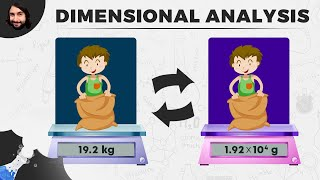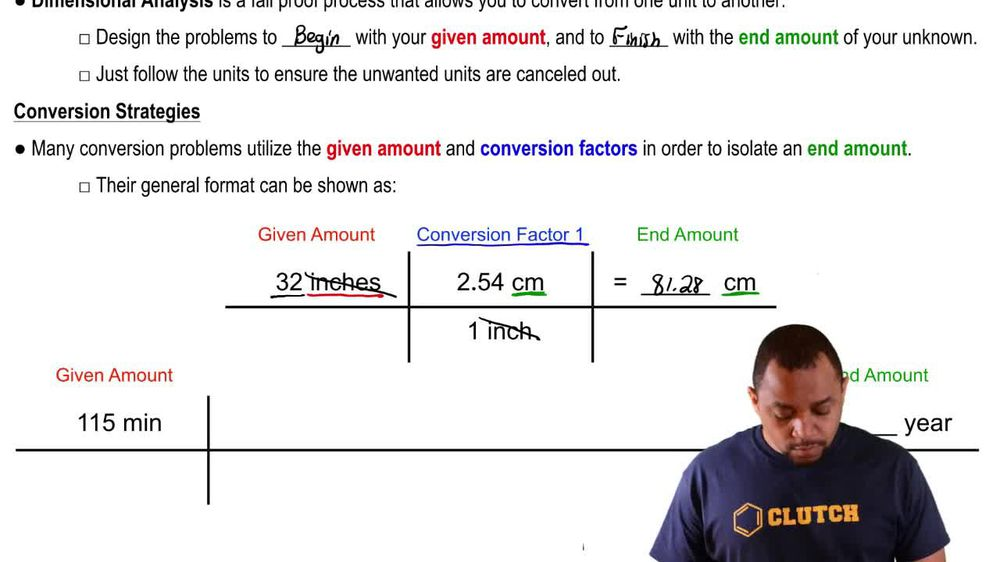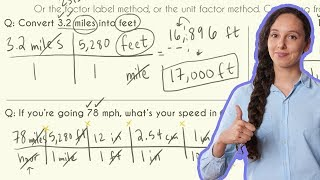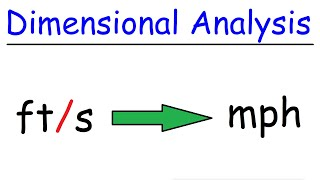Start typing, then use the up and down arrows to select an option from the list.
1. 1. Intro to General Chemistry2. Dimensional Analysis
Problem

# Among many alternative units that might be considered as a measure of time is the shake rather than the second. Based on the expression “faster than a shake of a lamb’s tail,” we’ll define 1 shake as equal to 2.5 * 10-4 s. If a car is traveling at 55 mi/h, what is its speed in cm/shake

Relevant Solution1m
Play a video:
Hey everyone, we're told that a blink can be used as an alternative unit for time rather than a second. If one blink is equal to 0.25 seconds, what is the speed of a train traveling at 300 km/h in yards per blink For this question? We're going to use dimensional analysis starting off with our 300 km per one hour. We want to convert our kilometers into yards using a conversion factor that we've learned. We know that we have 1,094 yards per one km Next, converting our hours into minutes. We know that one hour contains 60 minutes next. We will use our minutes to convert this into seconds and we know that one minute contains 60 seconds. And we were told in our questions, um, that 0.25 seconds Is equivalent to one blink. So when we calculate this out and cancel out our units, We end up with a total of 23 yards per blink. And this is going to be our final answer. Now, I hope that made sense. And let us know if you have any questions